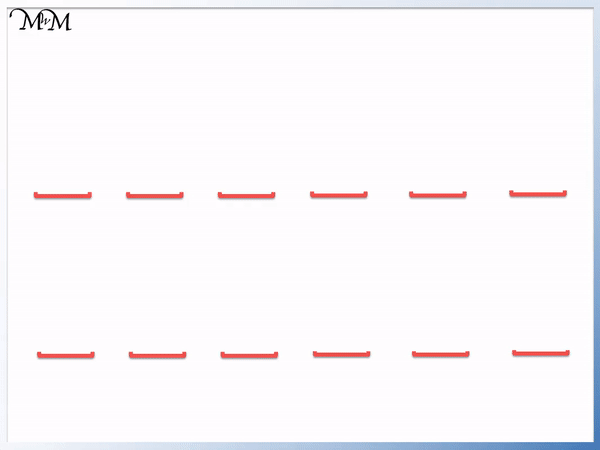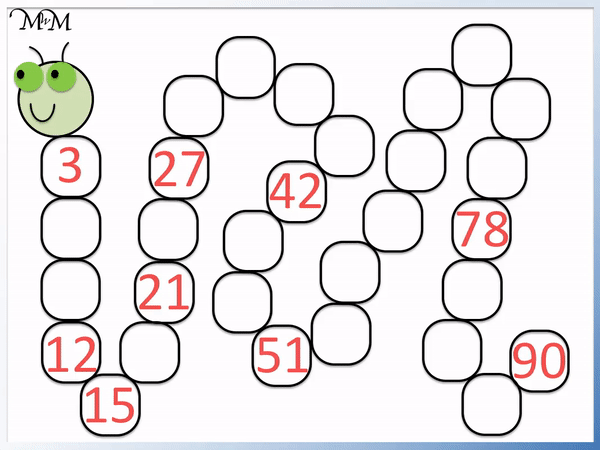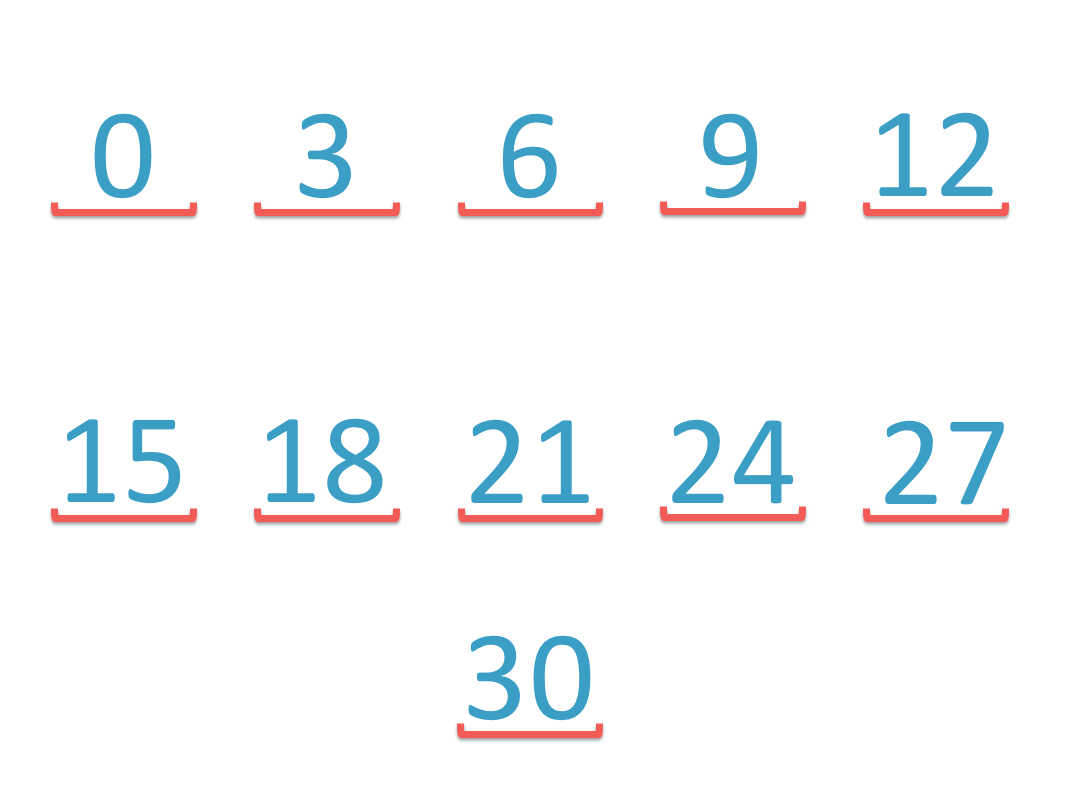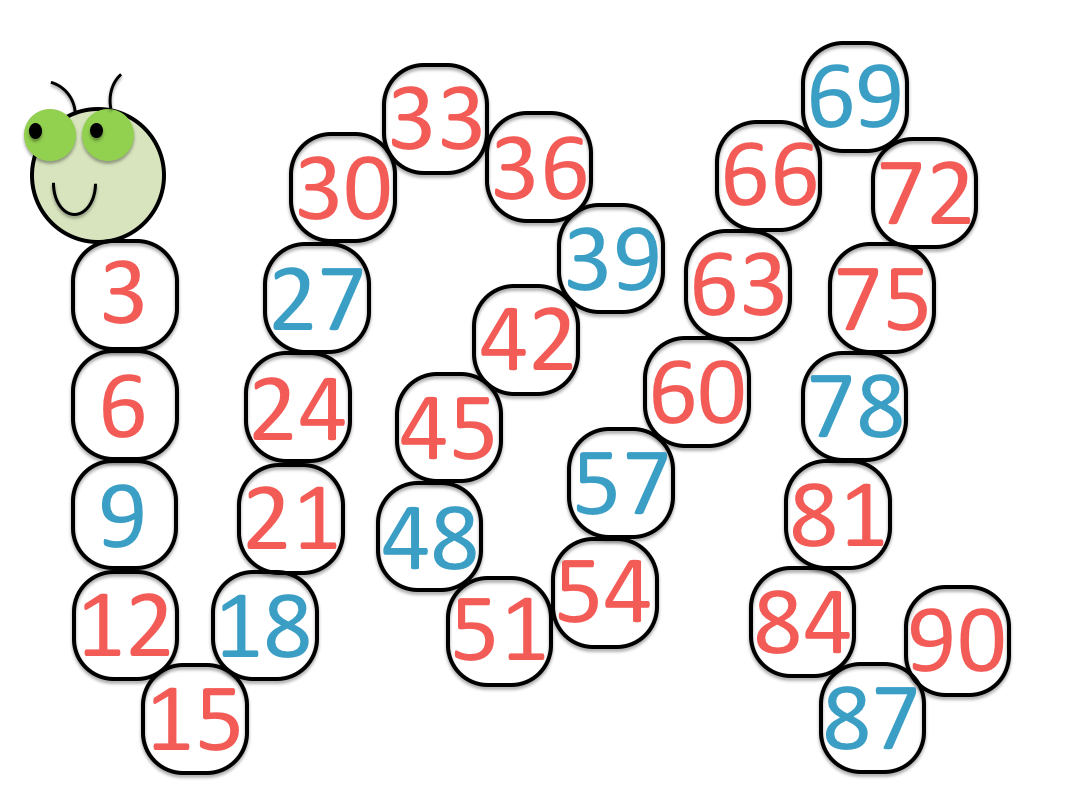# Skip Counting by 3

Skip Counting By 3• To skip count by 3 keep adding three to get to the next number.
• We can see diagonal patterns on the number grid when skip counting by 3.
• To skip count by 3 using the grid, we miss out two numbers and say the third.
• Skip counting in threes from zero is important for learning the three times table.
• We have 3, 9, 12, 15, 18, 21, 24, 27, 30 and so on.

To skip count by 3, add three each time to get to the next number.• To skip count by 3, add three to get to the next number.
• Starting at zero and adding 3 we have: 3, 6, 9, 12, 15, 18, 21, 24, 27 and 30.
• Once we get to 30 we have a number ending in 0.
• Adding 3 to 30 is similar to adding 3 to 0, we get 33 which ends in a 3.
• Learning the skip counting by 3 pattern up to 30 can help with counting on in threes with larger numbers.
• Adding 3 to a number ending in 9 produces a number ending in 2.
• Adding 3 to a number ending in 8 produces a number ending in 1.
• Adding 3 to a number ending in 7 produces a number ending in 0.#### Skip Counting by 3: Accompanying Lesson Worksheet# Skip Counting by 3

## What is Skip Counting by 3?

Skip counting by 3 means to count up in threes rather than ones. To get from one number to the next, simply add three each time. You skip out 2 numbers each time and only count the 3rd number.

Skip counting is a faster way to count than simply counting up in ones.

Skip counting by 3 means to add three to get to the next number, skipping two numbers out in the process.

Skip counting up in threes from zero we have:

0, 3, 6, 9, 12, 15, 18, 21, 24, 27, 30 …

Since there is not a clear pattern, a number grid can be a helpful teaching tool for teaching skip counting by three.When teaching skip counting by three using a number grid, it can be helpful to first shade or colour in the numbers before counting them.

With a number grid, it is easy to see which numbers are coming next and to see that we are skipping out two numbers each time.

Before looking at skip counting in threes with larger numbers we will look at the numbers up to 30 to see a pattern in the digits.Adding three each time from zero, we get the 3 times table:

0, 3, 6, 9, 12, 15, 18, 21, 24, 27, 30

To notice a pattern in the three times table, we can look at the digit in the units column for these numbers:

0, 3, 6, 9, 12, 15, 18, 21, 24, 27, 30

With 30 we are back to a units digit of ‘0’.

In the same way that 0 + 3 = 3, 30 + 3 = 33.The following numbers will have the same digit in the units column as those just seen.

In the first ten numbers, we had:

0, 3, 6, 9, 12, 15, 18, 21, 24, 27, 30

Continuing to add three, we get:

30, 33, 36, 39, 42, 45, 48, 51, 54, 57, 60

We have the same original ten numbers but we have added ’30’ to each of them.

When teaching skip counting by three, simply learning the first ten numbers first will help to learn the next set.

Adding a ‘3’ to the tens column of the digits above, we get:

60, 63, 66, 69, 72, 75, 78, 81, 84, 87, 90

The trickiest part of adding 3 is when we cross each multiple of 10.

It is worth teaching the following patterns in skip counting by 3.

• Adding three to a number ending in 9 results in a number ending in 2.

For example: 9 + 3 = 12, 39 + 3 = 42 and 69 + 3 = 72.

• Adding three to a number ending in 8 results in a number ending in 1.

For example: 18 + 3 = 21, 48 + 3 = 51 and 78 + 3 = 81.

• Adding three to a number ending in 7 results in a number ending in 0.

For example: 27 + 3 = 30, 57 + 3 = 60 and 87 + 3 = 90.

We can use these tips for skip counting by 3 to complete the following skip counting caterpillar.He can complete the skip counting caterpillar worksheet by adding three to each number and counting up.We will now look at skip counting backwards in threes.

When teaching skip counting, it is first best to introduce skip counting forwards. Once familiar with the numbers, skip counting backwards is useful for practising the concept.

To skip count backwards by 3, subtract three from each number. We keep subtracting three until we get to zero.Starting at 30 and subtracting three we get:

30, 27, 24, 21, 18, 15, 12, 9, 6, 3 and 0.We can practise skip counting backwards using a skip counting caterpillar worksheet shown below.

We start at 90 and subtract three from each number to count down in threes.Here is the completed skip counting caterpillar worksheet.You can download and print these skip counting caterpillar worksheets as PDF files above.Now try our lesson on Multiplication as Equal Groups where we learn how multiplication works.error: Content is protected !!